# Energies and powers (mechanical system)

## Introduction

This section deals with the stored energy and the power dissipated in a mechanical system in motion.

## Power: definition (reminder)

The instantaneous power developed by a force F (a torque G) is equal to the product of the values of the force (of the torque) and the instantaneous velocity.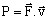(translating motion)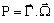(rotating motion)

## Regarding exerted forces

Among the forces which are exerted upon the mechanical system, one can distinguish:

• the conservative forces, which derive from a potential energy (see the following section)
• the non conservative forces, which can be:
• the forces of system interaction with an external operator, which can produce an increase or a decrease of the mechanical energy
• the forces caused by constraints (i.e. the forces of contact with another system, for example forces of friction if the object is moving in a fluid medium), which, by opposing the motion, yield a decrease (loss) of mechanical energy

## Elastic potential energy: definition

The potential mechanical energy of a mechanical system is the energy that the system possesses by:

• its position (potential energy of the gravity)
• its shape (elastic potential energy*)
Note: * By compressing a spring and then releasing it a rapid reversal to the original shape occurs, making it possible to propel an object. During the compression of the spring work is carried out over it, therefore; it accumulates elastic potential energy which can then be released as another form.

Example:

Let us consider a system consisting of a mass m subjected to the action of gravity and suspended from a spring of elastic constant k.

In this case, the system potential energy is equal to the sum:

• of a potential energy of gravity: mgx
• of a potential elastic energy: kx2 /2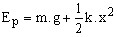## Stored energy

In the cases we are interested in the mechanical energy stored as the potential elastic energy. This is expressed as: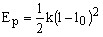(translating motion)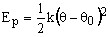(rotating motion)

## Dissipated power

The forces or torques that results in the dissipation of energy are the forces or torques yielded by the constraints (friction forces/torques).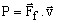(translating motion)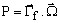(rotating motion)